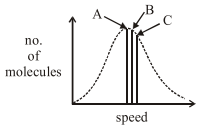# Solve this following

Question:

Identify the correct labels of A, B and C in the following graph from the options given below:Root mean square speed $\left(\mathrm{V}_{\mathrm{rms}}\right)$; most probable speed $\left(\mathrm{V}_{\mathrm{mn}}\right)$; Average speed $\left(\mathrm{V}_{\mathrm{ay}}\right)$

1. $\mathrm{A}-\mathrm{V}_{\mathrm{rms}} ; \mathrm{B}-\mathrm{V}_{\mathrm{mp}} ; \mathrm{C}-\mathrm{V}_{\mathrm{av}}$

2. $\mathrm{A}-\mathrm{V}_{\mathrm{av}} ; \mathrm{B}-\mathrm{V}_{\mathrm{rms}} ; \mathrm{C}-\mathrm{V}_{\mathrm{mp}}$

3. $\mathrm{A}-\mathrm{V}_{\mathrm{mp}} ; \mathrm{B}-\mathrm{V}_{\mathrm{rms}} ; \mathrm{C}-\mathrm{V}_{\mathrm{av}}$

4. $\mathrm{A}-\mathrm{V}_{\mathrm{mp}} ; \mathrm{B}-\mathrm{V}_{\mathrm{av}} ; \mathrm{C}-\mathrm{V}_{\mathrm{rms}}$

Correct Option: , 4

Solution:

\$V_{m p}\left(=\sqrt{\frac{2 R T}{M}}\right)